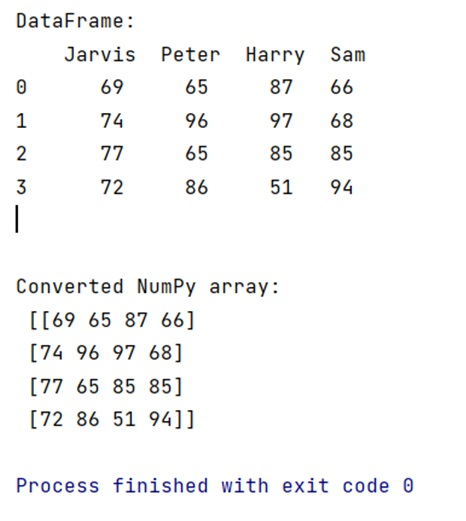# How to convert pandas DataFrame to NumPy array?

Pandas to NumPy Conversion: In this tutorial, we will learn how can we convert a pandas DataFrame to NumPy array with the help of example? By Pranit Sharma Last updated : April 18, 2023

## Overview

In python pandas, we mostly deal with a dataset in the form of DataFrame. DataFrames are 2-dimensional data structures in pandas. DataFrames consist of rows, columns, and the data. DataFrame can be created with the help of python dictionaries. Pandas also allow us to convert this DataFrame into a NumPy array using DataFrame.to_numpy() method.

## Pandas DataFrame to NumPy Array Conversion

To convert a Panda DataFrame to NumPy array, simply use the DataFrame.to_numpy() method which is called with the DataFrame that we want to convert and it returns a 2D NumPy array of the same size/dimensions.

### Syntax

```DataFrame.to_numpy(
dtype=None,
copy=False,
na_value=NoDefault.no_default
)
```

The dtype is the data type passed to the method, apart from dtype, it has two more optional parameters, i.e., copy and na_value.

Let us understand with the help of an example.

## Python program to convert pandas DataFrame to NumPy array

```# Importing pandas package
import pandas as pd

# Creating a dictionary of student marks
d = {
"Jarvis":[69,74,77,72],
"Peter":[65,96,65,86],
"Harry":[87,97,85,51],
"Sam":[66,68,85,94]
}

# Now, Create DataFrame
df = pd.DataFrame(d)

# Printing the DataFrame
print("DataFrame:\n",df,"\n\n")

# Converting the DataFrame into NumPy array
arr=df.to_numpy()

# Printing the array
print("Converted NumPy array:\n",arr)
```

### Output